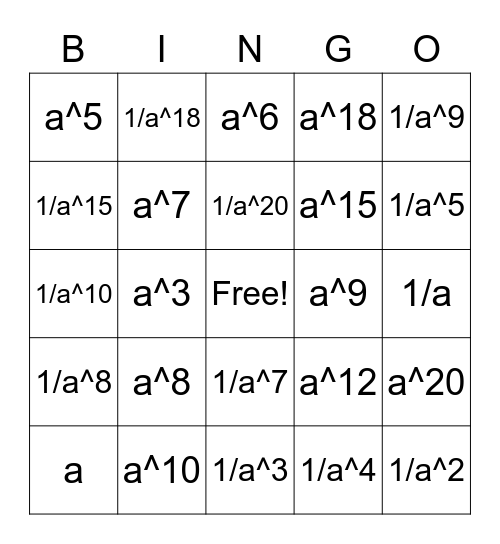# ExponentsThis bingo card has a free space and 29 words: a, a^2, a^3, a^4, a^5, a^6, a^7, a^8, a^9, a^10, 1/a, 1/a^2, 1/a^3, 1/a^4, 1/a^5, 1/a^6, 1/a^7, 1/a^8, 1/a^9, 1/a^10, a^12, 1/a^12, a^15, 1/a^15, a^18, 1/a^18, a^20, 1/a^20 and 1.

## Play Online